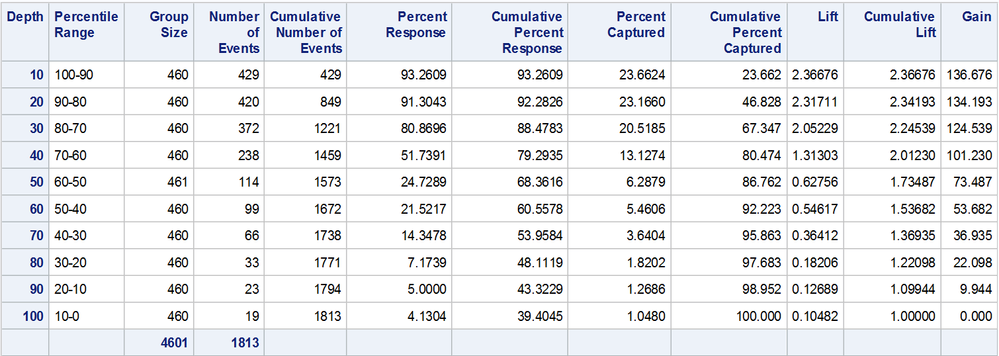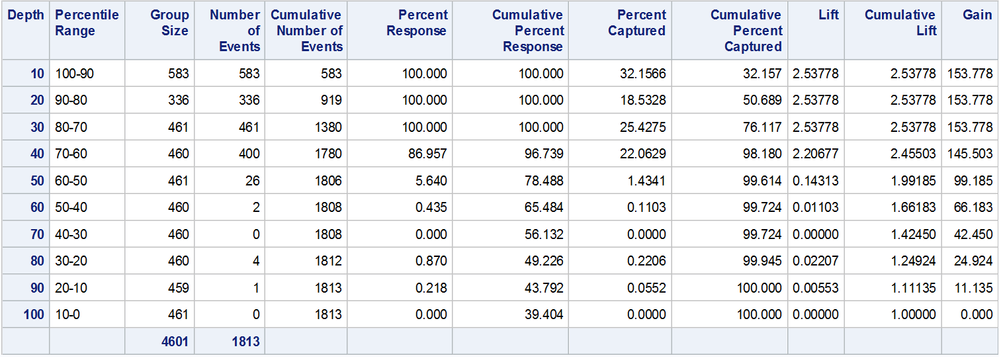## The %GAINLIFT macro (using PROC RANK) is not ranking data properly when there are many ties

I'm using 2 different procedures for binary classification: logistic regression (PROC LOGISTIC) and random forest (PROC HPFOREST).

First, consider PROC LOGISTIC.

``````proc logistic
data = sashelp.junkmail
noprint;
model	class (event = '1') = Exclamation Dollar Pound CapAvg CapLong CapTotal;
output	out = j1
p = P_Class1;
run;``````

I will now use the %GAINLIFT macro to produce the lift table.

http://support.sas.com/kb/41/683.html

``````%include 'Desktop\gainlift.sas';

%GainLift(data = j1, response = Class, p = P_Class1, event = '1', groups = 10, graphopts = NOGRAPH);``````

Here is the resulting table.Notice that the number of records in each decile group is roughly the same (n = 460 in most classes).

Now, let's consider PROC HPFOREST.

``````ods exclude all;
proc hpforest
data = sashelp.junkmail
seed = 123;
target 	Class / level = binary;
input 	Exclamation Dollar Pound CapAvg CapLong CapTotal / level = interval;
score 	out = j2;
run;
ods exclude none;

%GainLift(data = j2, response = Class, p = P_Class1, event = '1', groups = 10, graphopts = NOGRAPH);``````

Here is the resulting table.Notice that the number of records in the first 2 deciles are very different from the rest.

I suspect that this occurs because there are ties in the predicted probabilities from a random forest.  The %GAINLIFT macro uses PROC RANK to rank the predicted probabilities, and I suspect that PROC RANK cannot deal with ties very well.

My actual data set (which is confidential) shows much wider variation in the sample sizes in the decile groups than above.  This is a big problem, because I cannot use lift to assess the classifier when this occurs.

How can I modify the %GAINLIFT macro so that the resulting lift table has roughly equal sample sizes between the decile groups?

Thank you.

7 REPLIES 7

## Re: The %GAINLIFT macro (using PROC RANK) is not ranking data properly when there are many ties

Use the TIES option on the PROC RANK code to modify how it handles tied data. See the documentation for specifics.

http://documentation.sas.com/?docsetId=proc&docsetTarget=p16s2o8e4bnqrin1phywxdaxqba7.htm&docsetVers...

## Re: The %GAINLIFT macro (using PROC RANK) is not ranking data properly when there are many ties

Hi Reeza,

I tried TIES = HIGH, LOW, MEAN, and DENSE, and none of them produced equally large decile groups.

Does anybody else have any ideas?  I'm trying to use PROC UNIVARIATE to do the ranking, but I can't figure out how to incorporate it into the macro.

## Re: The %GAINLIFT macro (using PROC RANK) is not ranking data properly when there are many ties

Are you sure you want to? If you have too many ties that would indicate a different issue, possible imbalance in your data. I'm not sure expecting exactly equal groups is something that makes sense.

If the values are ties, how would you split them up?

Consider the series:

1

2

2

1

2

1

2

1

5

6

5

6

5

5

5

5

5

5

5

5

Trying to group the 5/6s with the 1/2s wouldn't make logical sense in this case.

My nickel of thoughts.

## Re: The %GAINLIFT macro (using PROC RANK) is not ranking data properly when there are many ties

Hi Reeza,

You may be right.  I may not want to break the ties.

My ultimate goal is to split the predicted probabilities from the random forest into equally large decile groups.  Perhaps ties are not the problem, but I don't know what else is causing the imbalance in the sample sizes.

Let's ignore the ties for now.  Given this problem with the macro for my predicted probabilities from the random forest, how should I solve it?

Thanks.

## Re: The %GAINLIFT macro (using PROC RANK) is not ranking data properly when there are many ties

Let's say that  for some reason, it's more important to generate same-size deciles that it is to preserve ties.

If so, then choose a very small epsilon to perturb p_class1 in tied groups prior to the GAINLIFT macro.   For each collection of tied values, add 0*epsilon to p_class1 for the 1st record,  add 1*epsilon to the second, 2*epsilon to the third, etc.

If the data are not absolutely pathological, you probably can find an epsilon small enough such that perturbing p_class1 for the last member of the largest tied group does not move p_class1 past the next distinct value.  And making such small changes in p_class1 should not change your substantive results if there is any real information in the data.  After all, the probabilities are estimates to begin with.

You might be able to set    epsilon=constant('small') or some multiple of constant('small').

--------------------------
The hash OUTPUT method will overwrite a SAS data set, but not append. That can be costly. Consider voting for Add a HASH object method which would append a hash object to an existing SAS data set

Would enabling PROC SORT to simultaneously output multiple datasets be useful? Then vote for
Allow PROC SORT to output multiple datasets

--------------------------

## Re: The %GAINLIFT macro (using PROC RANK) is not ranking data properly when there are many ties

@PurpleNinja wrote:

Hi Reeza,

You may be right.  I may not want to break the ties.

My ultimate goal is to split the predicted probabilities from the random forest into equally large decile groups.  Perhaps ties are not the problem, but I don't know what else is causing the imbalance in the sample sizes.

Let's ignore the ties for now.  Given this problem with the macro for my predicted probabilities from the random forest, how should I solve it?

Thanks.

DO NOT CALL THEM DECILES THEN. They also cannot be used to appropriately measure the differences because the groups are not actually different and any metrics will be uninterpretable.

But, if you want 10 equal groups, use a data step and END option to specify the number of groups.

This doesn't work well if you have small data but if you have a lot of data and bigger groups it's fine.

``````%let n_groups=5;

data want;
set sashelp.class nobs=n_total;
retain group_size;
if _n_ = 1 then do;
group_size=floor(n_total/&n_groups);
group_index=1;
end;

if _n_ > group_size*group_index then
group_index+1;

if group_index > &n_groups then group_index=&n_groups.;
run;
``````

## Re: The %GAINLIFT macro (using PROC RANK) is not ranking data properly when there are many ties

I think you are encountering the situation where you have tied values and you are trying to use quantiles to subdivide the data. In this case, you will not get evenly divided groups. For a general discussion and example, see the article "Binning data by quantiles? Beware of rounded data," which also suggests possible workarounds.

Discussion stats
• 7 replies
• 1472 views
• 0 likes
• 4 in conversation